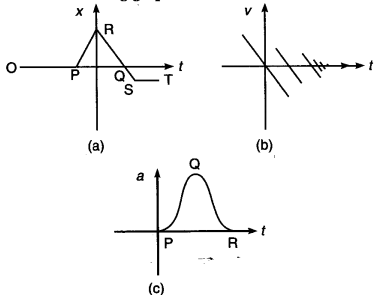# Suggest a suitable physical situation for each of the following graphs:

Suggest a suitable physical situation for each of the following graphs:(a) A ball at rest on a smooth floor is kicked OP, shows it rest position, at R the ball rebounds from the wall. RQ represents the bounced ball with reduced speed because the slope of RQ is less than that of PR. (The slope of x-t graph gives the speed of the moving body), ST shows the rest position of the ball.
(b) The graph shows the case of a ball thrown up with some initial velocity and rebounding from the floor with reduced speed after each hit every time on the floor, i.e., after every bounce.
© It is -the acceleration-time graph. The graph
represents a uniformly moving cricket ball turned back after hitting the bat for a very short time interval (represented by the portion PQR of the graph).